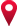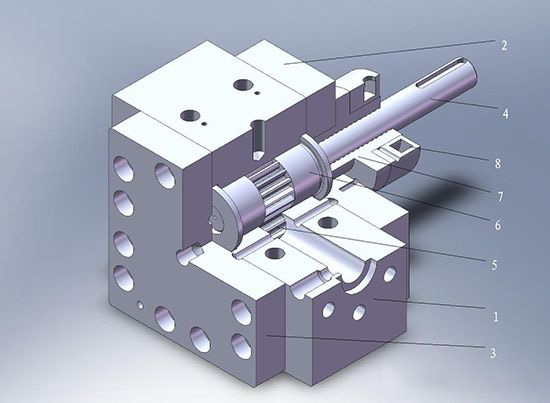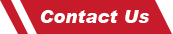# What is the relationship between melt pump displacement and gears?

For high temperature melt gear pumps, there are three standard gear types as well as some special gear types with smaller or wider gear pumps or adjusted geometries. High temperature polymer melt gear pumps are available in spur, helical and herringbone gears. Each of these gear types has different properties that are important to the use of the gear type. What is the relationship between melt pump displacement and gears?The volume of melt discharged by the melt pump per revolution is called the theoretical displacement of the pump, or the displacement of the pump, and the unit is cc/r. For a pump of a certain size, theoretically speaking, the volume of melt discharged by the pump per revolution is the same, so the size of the pump is generally characterized by the displacement. In practical engineering applications, a relatively simple formula is usually used to calculate the displacement of the melt pump. The formula is as follows: q=2πm2ZB (m is the modulus, Z is the number of teeth, and B is the width of the teeth). It can be known from the displacement formula that the displacement q is proportional to the number of teeth Z. When the module is constant, the number of teeth is large and the displacement is large. However, if the number of teeth is large, the volume of the melt pump increases. If the volume of the melt pump is kept unchanged, when Z increases, the displacement decreases. Therefore, the number of teeth should not be too many, generally 8-20 are appropriate.

When the melt pump in extruder displacement is constant, the number of spur gear teeth increases, and the overall size of the melt pump will increase, but the pressure pulsation will decrease. Therefore, when the flow pulsation of the melt pump is required, a larger number of teeth can be taken, generally 12-25. For a melt pump that focuses on the pressure buildup capacity, a smaller number of teeth can be taken, generally 8-14. However, the choice of the module and the number of teeth is a more complicated problem. In addition to the overall size and displacement, the width of the gear should also be considered. The width of the gear is limited. If the width of the gear is too narrow, the displacement will decrease; if the width of the gear is too large, the radial force will increase, and the load that the journal and bearing can bear will increase accordingly. Manufacturing difficulty also increases. In general, we use the concept of square gears, that is, the tooth width should be equal to the diameter of the index circle (B=mZ). At this time, the displacement formula of the melt pump can be sorted as: q= 2πm3Z2.Any questions about our products, please feel free to contact us! We promise you high-end products and first-class service.Look forward to our cooperation!!!